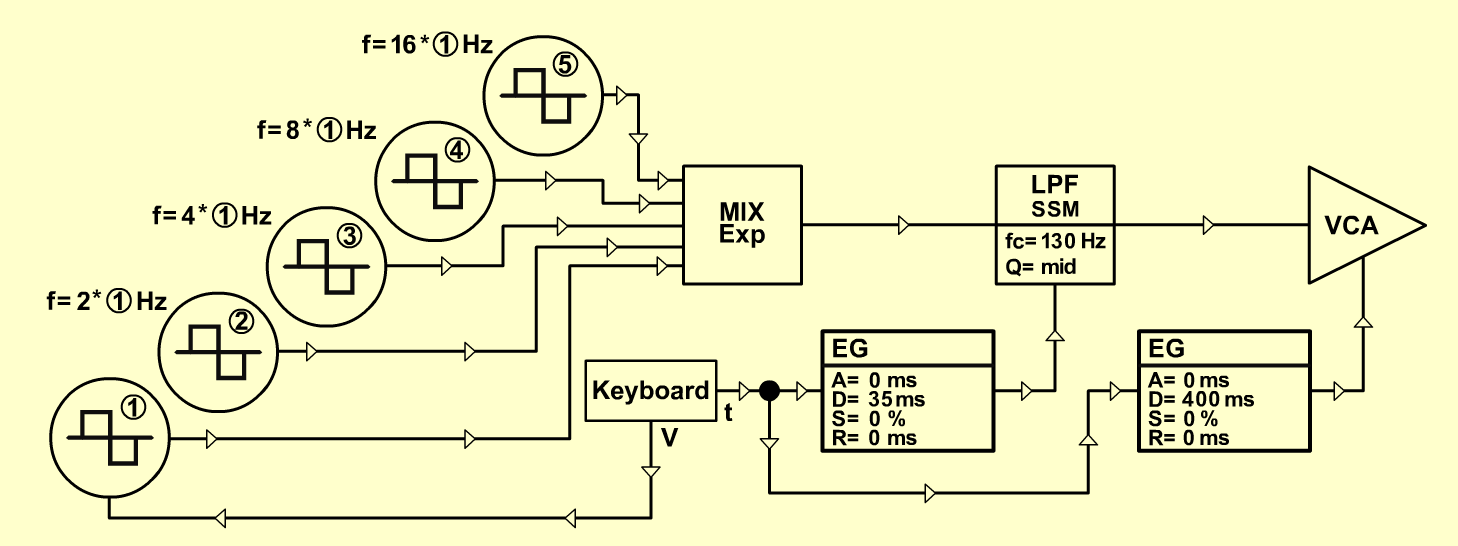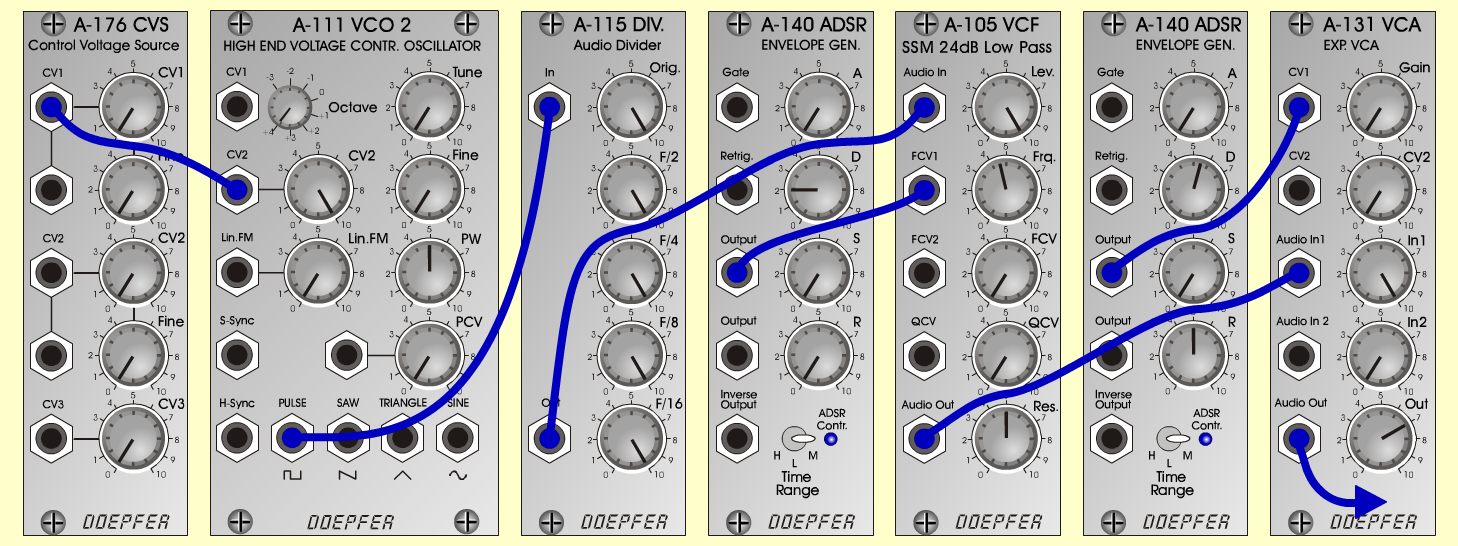Strings HitInstrumentsD o e p f e r A - 100 Connections: Settings: A-176 (CV1) <=> A-111 (CV2 A-111 (Pulse) <=> A-115 (I A-115 (Out) <=> A-105 (Audio I A-105 (Audio Out) <=> A-131 (Audio I A-140/1 (Output) <=> A-105 (FCV A-140/2 (Output) <=> A-131 (CV A-131 (Audio Out) <=> Amplifie) A-111 (Oct = +4, CV 2 = 10, PW = 5) A-115 (Orig = 10, F/2 = 10, F/4 = 10, F/8 = 10, F/16 = 10) A-105 (Lev = 10, Frq = 4.5, Res = 5) A-140/1 (A = 0, D = 2, S = 0, R = 0, Range = M) A-140/2 (A = 0, D = 5.5, S = 0, R = 0, Range = M) A-131 (Gain = 0, Audio In 1 = 10, Audio Out = 7) Notes: Change the timbre with the values of A-115. Josef MuellerSound samples Strings Hit chords Strings Hit (65 Hz) Strings Hit (261 Hz) Strings Hit (523 Hz) Strings Hit (1046 Hz)